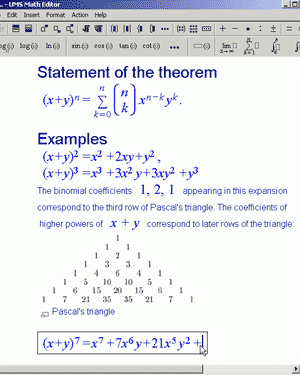UMS Math Editor

UMS Math Editor – New powerful formula editor

UMS Math Editor is the program used for typing plain text and mathematical formulas.
The distinctive feature of UMS Math Editor is that it not only allows you a simple way to enter mathematical formulas in a regular word processing environment, but also to work on solving them.
UMS Math Editor allows you to send all or part of the entered formula for solution or calculation to UMS software and, if desired, paste the solution (simplification, calculation, diagram, graph etc.) as a continuation of your typed text. Thus, you save time and effort.Z

You can convert UMS Math Editor files to MS Word files.

Z

You can save UMS Math Editor files as HTML files.

Z

You can work with Wikipedia. You can Copy/Paste formulas and text from Wikipedia pages into an UMS Math Editor file.

For Windows OS